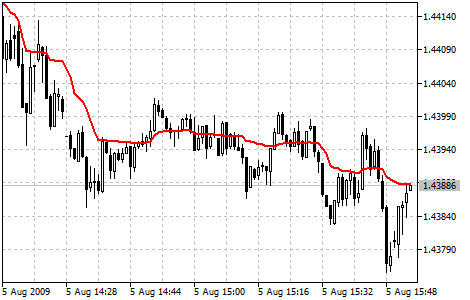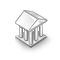Interesting script?
So post a link to it -
let others appraise it

You liked the script? Try it in the MetaTrader 5 terminalAdaptive Moving Average (AMA) - indicator for MetaTrader 5

Views:
18828
Rating:
Published:
2010.01.08 13:48
Updated:
2016.11.22 07:32

Adaptive Moving Average (AMA) is used for constructing a moving average with low sensitivity to price series noises and is characterized by the minimal lag for trend detection.

This indicator was developed and described by Perry Kaufman in his book "Smarter Trading".

One of disadvantages of different smoothing algorithms for price series is that accidental price leaps can result in the appearance of false trend signals. On the other hand, smoothing leads to the unavoidable lag in predicting the trends. This indicator was developed to overcome these two disadvantages.Adaptive Moving Average Indicator

Calculation:

To define the current market state Kaufman introduced the notion of Efficiency Ratio (ER), which is calculated by the below formula:

ER(i) = Sinal(i)/Noise(i)

where:

• ER(i) - current value of the Efficiency Ratio;
• Signal(i) = ABS(Price(i) - Price(i - N)) - current signal value, absolute value of difference between the current price and price N period ago;
• Noise(i) = Sum(ABS(Price(i) - Price(i-1)),N) - current noise value, sum of absolute values of the difference between the price of the current period and price of the previous period for N periods.

At a strong trend the Efficiency Ratio (ER) will tend to 1; if there is no directed movement, it will be a little more than 0.

The obtained value of ER is used in the exponential smoothing formula:

EMA(i) = Price(i) * SC + EMA(i-1) * (1 - SC)

where:

• SC = 2/(n+1) - EMA smoothing constant, n - period of the exponential moving;
• EMA(i-1) - previous value of EMA.

The smoothing ratio for the fast market mast be as for EMA with period 2 (fast SC = 2/(2+1) = 0.6667), and for the period of no trend EMA period must be equal to 30 (slow SC = 2/(30+1) = 0.06452). Thus the new changing smoothing constant is introduced (scaled smoothing constant) SSC:

SSC(i) = (ER(i) * ( fast SC - slow SC) + slow SC

or

SSC(i) = ER(i) * 0.60215 + 0.06425

For a more efficient influence of the obtained smoothing constant on the averaging period Kaufman recommends squaring it.

Final calculation formula:

AMA(i) = Price(i) * (SSC(i)^2) + AMA(i-1)*(1-SSC(i)^2)

or (after rearrangement):

AMA(i) = AMA(i-1) + (SSC(i)^2) * (Price(i) - AMA(i-1))

where:

• AMA(i) - current value of AMA;
• AMA(i-1) - previous value of AMA;
• SSC(i) - current value of the scaled smoothing constant.

Translated from Russian by MetaQuotes Software Corp.
Original code: https://www.mql5.com/ru/code/10Average Directional Movement Index Wilder

The Average Directional Movement Index by Wilder (ADX Wilder) helps to determine the presence of the price trend.Class CalcFrac - Number of bars before and after the current High / Low (calculated fractal)

Calculates fractals and lets you specify the number of bars separately before and after the current High / Low (fractal).Accumulation Swing Index (ASI)

ASI was created by Wales Wilder as an ordinary fluctuations indicator that gets signals from previous maximums and minimums of price.Average True Range (ATR)

Average True Range Technical Indicator (ATR) is an indicator that shows volatility of the market.